# 一类食饵具有常数收获率和Holling第II类功能性反应的捕食者–食饵模型的分支分析Bifurcation Analysis of a Predator-Prey Model with Constant Prey Harvesting Rate and Holling Type II Functional Response

DOI: 10.12677/DSC.2019.84029, PDF, HTML, XML, 下载: 328  浏览: 979

Abstract: In this paper, we study the equilibria and the bifurcation of a predator-prey model with constant prey harvesting rate and Holling type II functional response. Firstly, we analyze the existence condition of the equilibria of the system model. Then, we discuss the type and stability of the equilibria, Hopf bifurcation near positive equilibria and we obtain the conditions of Hopf bifurcation. Finally, the numerical simulation of the model is carried out and the relevant conclusions are obtained.

1. 引言

$\left\{\begin{array}{l}\frac{\text{d}x}{\text{d}t}=rx\left(1-\frac{x}{k}\right)-\frac{emxy}{a+x}-h,\\ \frac{\text{d}y}{\text{d}t}=y\left(\frac{mx}{a+x}-d\right).\end{array}$ (1)

$u=x,v=\frac{em}{r}y,\text{d}\tau =r\text{d}t,$

$\left\{\begin{array}{l}\frac{\text{d}u}{\text{d}\tau }=u\left(1-\frac{u}{k}\right)-\frac{uv}{a+u}-{h}_{0},\\ \frac{\text{d}v}{\text{d}\tau }=v\left(\frac{bu}{a+u}-c\right).\end{array}$ (2)

2. 平衡点类型及正平衡点的稳定性

1) 若 $v=0$，则有

${u}^{2}-ku+k{h}_{0}=0.$ (3)

$\Delta ={k}^{2}-4k{h}_{0}=k\left(k-4{h}_{0}\right)$

a) 当 $k=4{h}_{0}$ 时，则系统(2)有唯一边界平衡点 ${E}_{0}=\left(\frac{k}{2},0\right)$

b) 当 $k>4{h}_{0}$ 时，系统(2)有两个边界平衡点 ${E}_{1}=\left(\frac{k+\sqrt{\Delta }}{2},0\right)$${E}_{2}=\left(\frac{k-\sqrt{\Delta }}{2},0\right)$

2) 若 $v\ne 0$，则且当 $b>c$${h}_{0}<-\frac{u\left[ac-\left(b-c\right)k\right]}{k\left(b-c\right)}$ 时，系统(2)存在唯一的正平衡点 ${E}_{3}\left({u}^{*},{v}^{*}\right)$。其中， ${u}^{*}=\frac{ac}{b-c}$$J=\left(\begin{array}{cc}1-\frac{2u}{k}-\frac{av}{{\left(a+u\right)}^{2}}& -\frac{u}{a+u}\\ \frac{abv}{{\left(a+u\right)}^{2}}& \frac{bu}{a+u}-c\end{array}\right).$ (4)

1) 判断边界平衡点 ${E}_{0}$ 的类型

${E}_{0}=\left(\frac{k}{2},0\right)$ 代入Jacobi矩阵(4)中，可以得到系统(2)在 ${E}_{0}$ 处的Jacobi矩阵为

${J}_{{E}_{0}}=\left(\begin{array}{cc}0& -\frac{k}{k+2a}\\ 0& \frac{bk}{k+2a}-c\end{array}\right).$

2) 判断边界平衡点 ${E}_{1}$${E}_{2}$ 的类型

${E}_{1}=\left(\frac{k+\sqrt{\Delta }}{2},0\right)$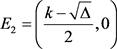代入Jacobi矩阵(4)中，可得到系统(2)在 ${E}_{1}$${E}_{2}$ 处的Jacobi矩阵为

${J}_{{E}_{1}}=\left(\begin{array}{cc}-\frac{\sqrt{\Delta }}{k}& -\frac{k+\sqrt{\Delta }}{2a+k+\sqrt{\Delta }}\\ 0& \frac{b\left(k+\sqrt{\Delta }\right)}{2a+k+\sqrt{\Delta }}-c\end{array}\right),\text{\hspace{0.17em}}{J}_{{E}_{2}}=\left(\begin{array}{cc}\frac{\sqrt{\Delta }}{k}& -\frac{k-\sqrt{\Delta }}{2a+k-\sqrt{\Delta }}\\ 0& \frac{b\left(k-\sqrt{\Delta }\right)}{2a+k-\sqrt{\Delta }}-c\end{array}\right)$

a) 若 $c>\frac{b\left(k+\sqrt{\Delta }\right)}{2a+k+\sqrt{\Delta }}$，那么 ${E}_{1}$ 为稳定结点；若 $c<\frac{b\left(k+\sqrt{\Delta }\right)}{2a+k+\sqrt{\Delta }}$，则 ${E}_{1}$ 为鞍点。

b) 若 $c<\frac{b\left(k-\sqrt{\Delta }\right)}{2a+k-\sqrt{\Delta }}$，那么 ${E}_{2}$ 为不稳定结点；若 $c>\frac{b\left(k-\sqrt{\Delta }\right)}{2a+k-\sqrt{\Delta }}$，则 ${E}_{2}$ 为鞍点。

3) 正平衡点 ${E}_{3}$ 的稳定性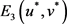带入Jacobi矩阵(4)中，可以得到系统(2)在 ${E}_{3}\left({u}^{*},{v}^{*}\right)$ 处的Jacobi矩阵为

${J}_{{E}_{3}}=\left(\begin{array}{cc}{f}_{1}\left({v}^{*}\right)& -\frac{c}{b}\\ \frac{{v}^{*}{\left(b-c\right)}^{2}}{ab}& 0\end{array}\right).$ (5)

$D=\mathrm{det}{J}_{{E}_{3}}=\frac{c{v}^{*}{\left(b-c\right)}^{2}}{a{b}^{2}},T=trace{J}_{{E}_{3}}={f}_{1}\left({v}^{*}\right)=1-\frac{2ac}{k\left(b-c\right)}-\frac{{v}^{*}{\left(b-c\right)}^{2}}{a{b}^{2}}$

$D>0$$T<0$ ；我们可得到系统(2)在正平衡点 ${E}_{3}\left({u}^{*},{v}^{*}\right)$ 处是局部渐近稳定的；

$D>0$$T>0$ ；我们可得到系统(2)在正平衡点 ${E}_{3}\left({u}^{*},{v}^{*}\right)$ 处是不稳定的。

${f}_{1}\left({v}^{*}\right)=0$，既有 $D>0$$T=0$ ；由参考文献 ，则Jacobi矩阵 ${J}_{{E}_{3}}$ 的特征方程有一对纯虚的特征根 $±i{\omega }_{0}$ (其中i为虚数单位， ${\omega }_{0}=\frac{b-c}{b}\sqrt{\frac{{v}^{*}c}{a}}>0$ )。

${E}_{3}\left({u}^{*},{v}^{*}\right)$ 代入 ${f}_{1}\left({v}^{*}\right)=0$ 中，解得 ${h}_{0}^{*}=\frac{a{c}^{2}\left[a\left(b+c\right)-k\left(b-c\right)\right]}{{\left(b-c\right)}^{3}k}$

a) 若 ${h}_{0}>{h}_{0}^{*}$，则系统(2)的正平衡点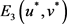是局部渐进稳定的；

b) 若 $0<{h}_{0}<{h}_{0}^{*}$，则系统(2)的正平衡点是不稳定的；

c) 若 ${h}_{0}={h}_{0}^{*}$，则系统(2)在正平衡点 ${E}_{3}\left({u}^{*},{v}^{*}\right)$ 处出现Hopf分支。

3. Hopf分支

${\lambda }^{2}-\lambda {f}_{1}\left({v}^{*}\right)+\frac{{v}^{*}c{\left(b-c\right)}^{2}}{a{b}^{2}}=0.$ (6)

$k{\left(b-c\right)}^{2}\left(cv+{h}_{0}b\right)+abc\left[ac-k\left(b-c\right)\right]=0.$

$f\left(v\right)=k{\left(b-c\right)}^{2}\left(cv+{h}_{0}b\right)+abc\left[ac-k\left(b-c\right)\right]$，则有 $f\left({v}^{*}\right)=0$${f}^{\prime }\left({v}^{*}\right)>0$

$f\left({v}^{*}\right)=0$ 解得 ${v}^{*}\left({h}_{0}\right)=-\frac{\left\{ac\left[ac-k\left(b-c\right)\right]+{\left(b-c\right)}^{2}{h}_{0}k\right\}b}{{\left(b-c\right)}^{2}kc}$，且有 ${v}^{*}{}^{\prime }\left({h}_{0}\right)=\frac{b}{c}>0$

$p\left({h}_{0}\right)=\frac{{f}_{1}\left({v}^{*}\right)}{2}$。于是有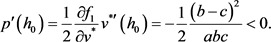${p}^{\prime }\left({h}_{0}^{*}\right)<0$ 可知系统(2)满足产生Hopf分支的条件 。因此系统(2)在 ${h}_{0}={h}_{0}^{*}$ 附近可产生Hopf分支。接下来我们来讨论产生的Hopf分支的方向。

${w}_{1}=u-{u}^{*}$${w}_{2}=v-{v}^{*}$。则系统(2)变成以下形式

$\left\{\begin{array}{l}\frac{\text{d}{w}_{1}}{\text{d}\tau }=-\frac{c}{b}{w}_{2}-\frac{{a}^{2}{b}^{3}-k{v}^{*}{\left(b-c\right)}^{3}}{{a}^{2}{b}^{3}k}{w}_{1}^{2}-\frac{{\left(b-c\right)}^{2}}{a{b}^{2}}{w}_{1}{w}_{2}-\frac{{v}^{*}{\left(b-c\right)}^{4}}{{a}^{3}{b}^{4}}{w}_{1}^{3}+\frac{{\left(b-c\right)}^{3}}{{a}^{2}{b}^{3}}{w}_{1}^{2}{w}_{2}+\cdots ,\\ \frac{\text{d}{w}_{2}}{\text{d}\tau }=\frac{{v}^{*}{\left(b-c\right)}^{2}}{ab}{w}_{1}-\frac{{v}^{*}{\left(b-c\right)}^{3}}{{a}^{2}{b}^{2}}{w}_{1}^{2}+\frac{{\left(b-c\right)}^{2}}{ab}{w}_{1}{w}_{2}+\frac{{v}^{*}{\left(b-c\right)}^{4}}{{a}^{3}{b}^{3}}{w}_{1}^{3}-\frac{{\left(b-c\right)}^{3}}{{a}^{2}{b}^{2}}{w}_{1}^{2}{w}_{2}+\cdots .\end{array}$ (7)

${\omega }_{0}=\frac{b-c}{b}\sqrt{\frac{{v}^{*}c}{a}}$，再作变换：

$\xi =-\frac{{v}^{*}{\left(b-c\right)}^{2}}{ab}{w}_{1},\eta =-{\omega }_{0}{w}_{2},$

$\left\{\begin{array}{l}\frac{\text{d}\xi }{\text{d}\tau }=-{\omega }_{0}\eta +\frac{{a}^{2}{b}^{3}{\xi }^{2}-k{v}^{*}{\left(b-c\right)}^{3}{\xi }^{2}}{a{b}^{2}{v}^{*}k{\left(b-c\right)}^{2}}+\frac{{\left(b-c\right)}^{2}\xi \eta }{a{b}^{2}{\omega }_{0}}+\frac{{\xi }^{3}}{a{b}^{2}{v}^{*}}+\frac{\left(b-c\right){\xi }^{2}\eta }{a{b}^{2}{v}^{*}{\omega }_{0}}+\cdots ,\\ \frac{\text{d}\eta }{\text{d}\tau }={\omega }_{0}\xi +\frac{{\omega }_{0}{\xi }^{2}}{{v}^{*}\left(b-c\right)}-\frac{\xi \eta }{{v}^{*}}+\frac{{\omega }_{0}{\xi }^{3}}{{v}^{*}{}^{2}{\left(b-c\right)}^{2}}-\frac{{\xi }^{2}\eta }{{v}^{*}{}^{2}\left(b-c\right)}+\cdots .\end{array}$ (8)

$\frac{\text{d}z}{\text{d}\tau }=i{\omega }_{0}z+\underset{2\le k+l\le \text{3}}{\sum }{g}_{kl}{z}^{k}{\stackrel{¯}{z}}^{l},$

${g}_{20}=\frac{1}{4}\left[\frac{ab}{{v}^{*}k{\left(b-c\right)}^{2}}-\frac{b-c}{a{b}^{2}}-\frac{1}{{v}^{*}}\right]+\frac{1}{4}i\left[\frac{{\omega }_{0}}{{v}^{*}\left(b-c\right)}-\frac{{\left(b-c\right)}^{2}}{a{b}^{2}{\omega }_{0}}\right],$

${g}_{11}=\frac{1}{2}\left[\frac{ab}{{v}^{*}k{\left(b-c\right)}^{2}}-\frac{b-c}{a{b}^{2}}\right]+\frac{1}{2}i\frac{{\omega }_{0}}{{v}^{*}\left(b-c\right)},$

${g}_{21}=\frac{1}{8{v}^{*}}\left[\frac{3}{a{b}^{2}{v}^{*}}-\frac{1}{{v}^{*}\left(b-c\right)}\right]+\frac{1}{8{v}^{*}}i\left[\frac{3{w}_{0}}{{v}^{*}{\left(b-c\right)}^{2}}-\frac{b-c}{a{b}^{2}{\omega }_{0}}\right].$

${l}_{1}\left(0\right)=\frac{1}{2{\omega }_{0}^{2}}\mathrm{Re}\left(i{g}_{20}{g}_{11}+{\omega }_{0}{g}_{21}\right),$

${l}_{1}\left(0\right)=\frac{1}{8{\omega }_{0}}\left\{\frac{1}{2a{b}^{2}{\omega }_{0}^{2}}\left[\frac{ab{v}^{*}+5}{{v}^{*}{}^{2}k}-\frac{{\left(b-c\right)}^{3}}{a{b}^{2}}\right]-\frac{ab}{{v}^{*}{}^{2}{\left(b-c\right)}^{3}k}\right\}.$

a) 当 ${l}_{1}\left(0\right)<0$ 时，模型(1)在  附近可以产生超临界的Hopf分支；

b) 当 ${l}_{1}\left(0\right)>0$ 时，模型(1)在 ${E}_{3}$ 附近可以产生次临界的Hopf分支。

4. 仿真算例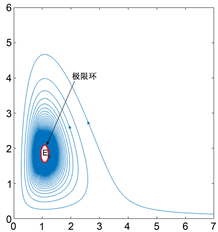(a)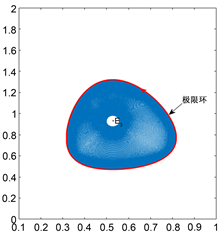(b)

Figure 1. (a) When $a=1.8,b=3.2,c=1.2,k=3.6,{h}_{0}=0.066$, there is a stable limit cycle near ${E}_{3}$ ; (b) When $a=1.9,b=2.3,c=0.5,k=1.5,{h}_{0}=0.14$, there is a unstable limit cycle near ${E}_{3}$

5. 结论

  马知恩. 种群生态学的数学建模与研究[M]. 合肥: 安徽教育出版社, 1996.  Zhu, C.R. and Lan, K.Q. (2010) Phase Portraits, Hopf Bifurcation and Limit Cycles of Leslie-Gower Predator-Prey Systems with Harvesting Rates. Discrete and Continuous Dynamical Systems—Series B, 14, 289-306. https://doi.org/10.3934/dcdsb.2010.14.289  Gong, Y.J. and Huang, J.C. (2014) Bogdanov-Takens Bifurcation in a Leslie-Gower Predator-Prey Model with Prey Harvesting. Acta Mathematicae Applicatae Sinica, English Series, 30, 239-244. https://doi.org/10.1007/s10255-014-0279-x  刘宣亮, 王志星. 一类捕食者有传染病的捕食者-食饵模型的分支分析[J]. 北华大学学报(自然科学版), 2018, 19(3): 281-290.  梁肇军, 陈兰荪. 食饵种群具有常数收获率的二维volterra模型的定性分析[J]. 生物数学报, 1986(1): 22.  傅仙发, 陈剑峰, 岳金健. 食饵带收获率的Holling-2型捕食者——食饵模型的Bautin分岔[J]. 长春师范大学学报, 2018, 37(12): 1-3.  张攀. Holling II型捕食者——食饵模型的稳定性和Hopf分支[J]. 洛阳理工学院学报(自然科学版), 2019(1): 83-85.  Wang, X.Y., Zanette, L. and Zou, X.F. (2016) Modelling the Fear Effect in Predator-Prey Interactions. Journal of Mathematical Biology, 73, 1179-1204. https://doi.org/10.1007/s00285-016-0989-1  Mukhopadhyay, B. and Bhattacharyya, R. (2016) Effects of Harvesting and Predator Interfererce in a Model of Two-Predators Competing for a Single Prey. Applied Mathematical Modelling, 40, 3264-3274. https://doi.org/10.1016/j.apm.2015.10.018  张芷芬, 丁同仁, 黄文灶, 董镇喜. 微分方程定性理论[M]. 北京: 科学出版社, 1985.  马知恩, 周义仓. 常微分方程定性与稳定性方法[M]. 北京: 科学出版社, 2007.  Peng, R. and Wang, M. (2007) Global Stability of the Equilibrium of a Diffusive Holling-Tanner Prey-Predator Model. Applied Mathematics Letters, 20, 664-670. https://doi.org/10.1016/j.aml.2006.08.020  Kuznetsov, Y.A. (1998) Elements of Applied Bifurcation Theory. 2nd Edition, Springer-Verlag, New York.